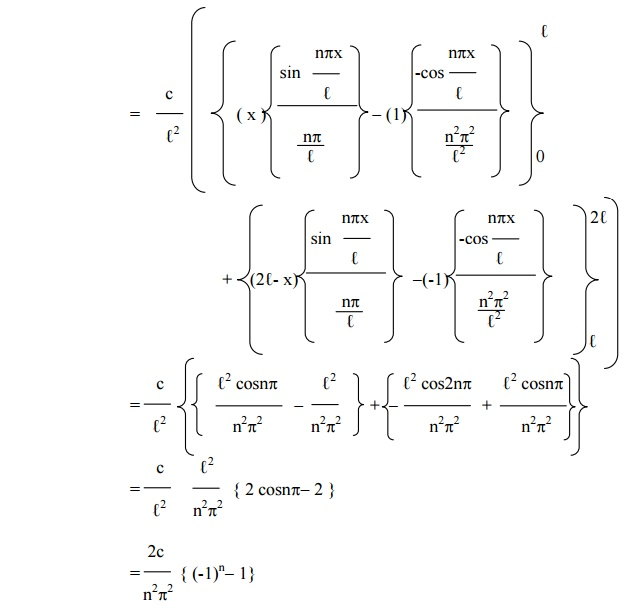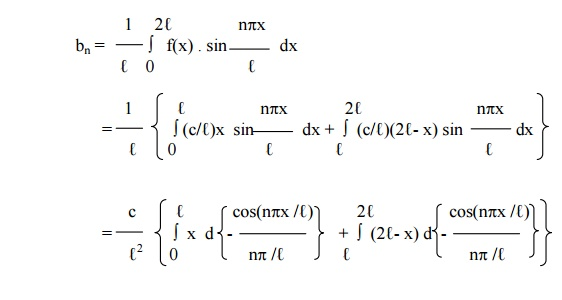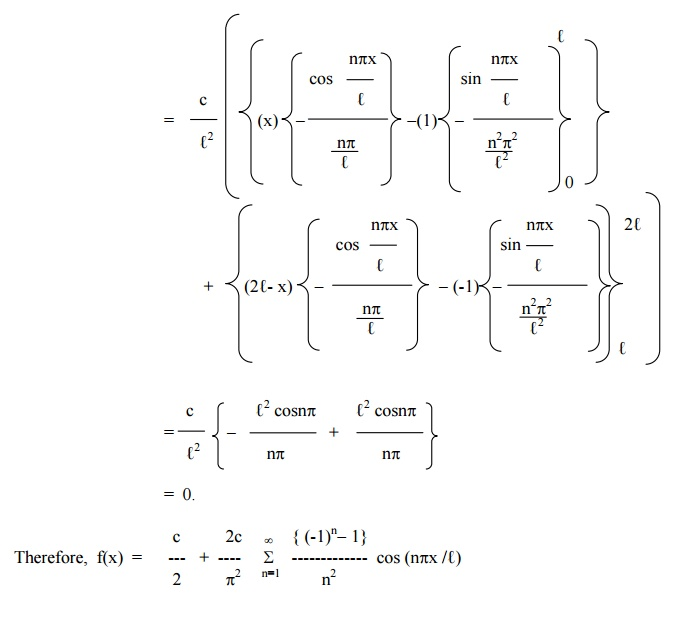Home | | Transforms and Partial Differential Equations | ParsevalŌĆÖs Theorem and Change of Interval

# ParsevalŌĆÖs Theorem and Change of Interval

The use of r.m.s value of a periodic function is frequently made in the theory of mechanical vibrations and in electric circuit theory. The r.m.s value is known as the effective value of the function.

ParsevalŌĆÖs Theorem

Root Mean square value of the function f(x) over an interval (a, b) is defined asThe use  of r.m.s value of a periodic  function is frequently made in the theory of mechanical vibrations and in electric circuit theory. The r.m.s value  is known as the effective value of the  function.

ParsevalŌĆÖs   Theorem

If f(x) defined in the interval (c, c+2ŽĆ ), then the ParsevalŌĆ¤s Identity is given byExample 13

Obtain the Fourier series for f(x)CHANGE OF INTERVAL

In most of the Engineering applications, we require an expansion of a given function over an interval 2l other than 2p.

Suppose f(x) is a function defined in the interval c< x < c+2l. The Fourier expansion for f(x) in the interval c<x<c+2l is given byEven and Odd Function

If f(x) is an even function and is defined in the interval ( c, c+2 l ), thenHalf Range Series

Sine SeriesCosine seriesExample 14

Find the Fourier series expansion for the functionExample 15

Find the Fourier series of periodicity 3 for f(x) = 2x ŌĆōx2 , in 0 <<3.

Here 2Ōäō = 3.

\ Ōäō = 3 / 2.Exercises

1.Obtain the Fourier series for f(x) = px in 0 < x < 2. 2.Find the Fourier series to represent x2 in the interval (-l, l ). 3.Find a Fourier series in (-2, 2), if

f(x) = 0, -2 < x < 0

= 1, 0 < x < 2.

4.Obtain the Fourier series for

f(x) =          1-x in 0 < x < l

= 0 in  l      < x < 2 l.     Hence deduce that

1- (1/3 ) +(1/5) ŌĆō(1/7) p/4+&  ŌĆ”   =

2                2        ) + (1/5        2                 2

(1/1 ) + (1/3 )   +  pŌĆ”/8) =   (

5.If f(x) = px,                          0 < x < 1

p (2-x), 1 < x < 2,

Show that in the interval (0,2),6.Obtain the Fourier series for

f(x) = x in 0 < x < 1

= 0 in 1 < x < 2

7.Obtain the Fourier series for

f(x) =          (cx /l ) in 0 < x < l

=       (c/l  ) (2 l  - x ) in l          < x < 2 l .

8.Obtain the Fourier series for

f(x) = (l + x ), - l < x < 0. = (l - x ), 0 < x < l.10.Express f(x) = x  as a half ŌĆōrange sine series in 0 < x < 2

11.Obtain the half-range  sine series for ex in 0 < x < 1.

12.Find the half ŌĆōrange cosine series for the function f(x) = (x-2)2 in the interval 0 < x < 2.

Study Material, Lecturing Notes, Assignment, Reference, Wiki description explanation, brief detail
Mathematics (maths) : Fourier Series : ParsevalŌĆÖs Theorem and Change of Interval |